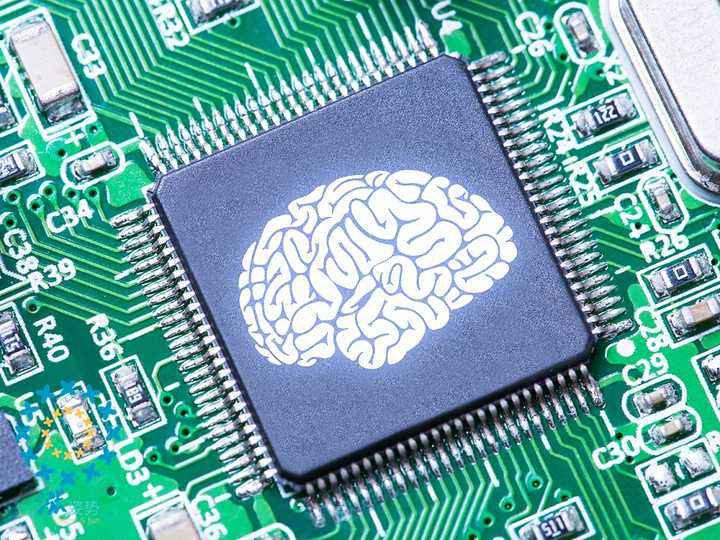# Why Do Computers Use Binary Numbers

By Math Is Fun, July 16, 2022

This is the third episode of the Binary Numbers And Computers series. In Episode I, we introduced binary numbers, which are used by Computers. In Episode II, we explained why humans use decimal numbers.

In this episode, we are going to dig a little deeper to see why binary numbers are so important to computers.

“Do you know why computers were invented in the first place?” I asked my son.

“Why?”

“Modern computers were invented in World War II. They were created to perform mathematical calculations much faster than humans. For example, the US created a computer to calculate missile trajectories. The Britishes, led by Alan Turing, created a computer to crack encrypted codes by the Germans.”

“So how do computers perform calculations?”

“Right, that’s what we are going to talk about today.”

Computers use binary numbers because electronics have two states: on and off. It turns out, basic binary calculations can be achieved by the way the electric circuits are wired.

Let’s start by a mathematical operation called AND. It’s also a logical operation. These are the results when performing the AND operation on two single digit binary numbers (a, b).

Very importantly, when a and b are single digit binary numbers, the result of a AND b is exactly the result of a * b. (a multiplied by b). So how is the AND operation performed in an electric circuit?

This is called a series circuit. In the picture, we have a battery, two switches and a light bulb.

• The switches represent the input a and b respectively. When the switch is off, it represents the digit 0. When the switch is on, it represents the digit 1.
• The light bulb represents the result of (a AND b). When the light is off, the digit is 0. When the light is on, the digit is 1.

Now you can see that the series circuit completes exactly the AND operation! Only when both inputs are 1 (a.k.a. Both switches are on), the output is 1 (a.k.a. The light is on). In all other cases, the output is 0 – the light is off. Since a and b are single digit binary numbers, the result of a AND b is exactly the result of a * b. (a multiplied by b). Of course, multiplication of multi-digit binary numbers is more complicated, but you can see how series circuits form the basis for multiplication operations.

Another common binary/logical operation is called OR. Here are the results for single digit binary numbers performing the OR operation.

The electric circuit to perform this operation is called a parallel circuit.

In this picture, if any of the switches (inputs) is 1, the light is on.

Can the addition of two single-digit binary numbers be performed with the OR operation? Not really. When a=1 b=1, without considering the carry digit, a + b = 0. That’s different from the OR operation. To perform an addition (without considering the carry digit), we need to use the XOR operation.

How is XOR done in an electric circuit?

This needs a little explanation:

• When both switches are off (0), obviously the light is off (0).
• When one of the switches is on, the battery power next to the switch can go through the circuit and the light is on (1).
• When both switches are on, both battery powers can go through the circuit, but since the batteries are in opposite directions, they cancel each other. The light receives net zero power so it remains off.

Now we have shown you electric circuits can be wired differently to perform simple binary operations. This is the basis for how computers work! To perform more complex operations, you just need more circuits! The whole idea of computer chips is basically to make the circuits so small, that we can fit more and more circuits into smaller and smaller chips! The unit in modern chips is called a transistor. Nowadays, a small chip can contain 10 billion transistors.